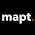# Memory Measurements Units in Computer

Different Physical quantities has different aspects of measurement.For example, Length is measured in Centi-meter/Meter/Kilometer.

Computer also has its memory and storage sizes measurement units.

What is the memory measurement unit of Computer?

You all know that a computer only understands the binary language.The information stored in the computer is in the form of 0`s and 1`s called bits.
Now the point is What is a bit ( Binary digit )?

A bit is the smallest unit of the data/information.A bit can have only a single value either 0 or 1.A group of 8-bits makes 1 Byte.

## Different Computer Memory Units and Relation between them :

4 bits =1 nibble
8 bits = 1 Byte
1024 Bytes = 1 KiloByte
1024 KiloBytes = 1 MegaByte
1024 MegaBytes = 1 GigaByte
1024 GigaBytes = 1 TeraByte
1024 TeraBytes = 1 PetaByte
1024 PetaByte = 1 ExaByte
1024 ExaByte = 1 ZettaByte
1024 ZettaByte = 1 YottaByte

bit=Binary digit     Byte=B     KiloByte=KB     MegaByte=MB     GigaByte=GB

TeraByte=TB     PetaByte=PB     ExaByte=EB     ZettaByte=ZB

YottaByte=YBHave Fun!

1.2.3.It is very helpful for computer thankz

4.5.Bit
Byte
Kb
Mb
Gb
Tb
Pb
Eb
Zb
Yb
Bb Boronto Byte
GeOP pronounce as GOP

About | Contact | Disclaimer-Privacy Statement